# VMC PL Math Sequencer Design

## PID Controller Design with Model Composer for Versal ACAPs (XAPP1376)

Document ID
XAPP1376
Release Date
2022-03-09
Revision
1.0 English

It is possible to use C or C++ to describe a control algorithm while concurrently changing the algorithm on the fly. For example, PID, PI, or lead-lag type controllers can be described using C and C++. Control algorithms are simply a series of arithmetic operations such as multiplication, addition, subtraction, saturation, and division. C and C++ easily describe a state machine that has memory (for intermediate data and instruction storage), inputs (like the W and Y inputs needed for the PID controller example), math operators, and output (PWM duty cycle) that are used to drive a DAC to control a servo motor. The arrival of input data, like W and Y inputs for the PID control loop, can be used to kick off the math sequence functionality while the end of the math sequence could be used to update a PWM. The following figure provides an example of a math sequencer optimized for a PID algorithm.

Figure 1. Math Sequencer Block Diagram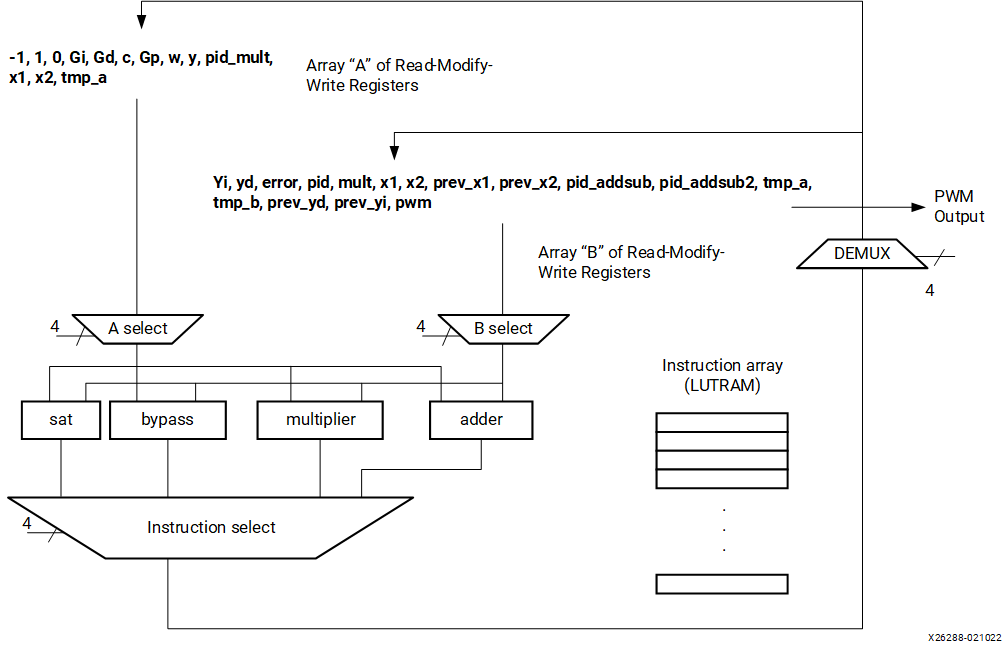An instruction array stored in LUTRAM controls the A and B multiplexer selects, where the resulting arithmetic operation needs to be stored in read-modify-write registers. The instruction array also controls what specific SPFP arithmetic operation (saturation, bypass, multiply, or add) is required. An instruction bit breakdown for the proposed math sequencer is shown in the following figure.

Figure 2. Math Sequencer Instruction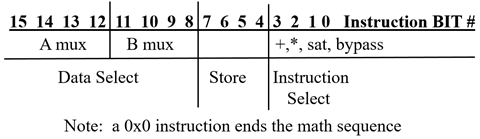There are ways to reduce the amount of arithmetic hardware needed at the expense of clock cycles. For example, y = (a – b) would require a two step operation using b × –1 -> b (b is multiplied by –1 and then stored into register b), followed by a second operation of a + b -> b. The results of an arithmetic operation is stored in either the A or B register array, which is not desirable for the next operand input needed to follow the arithmetic operation. To work around these issues, a bypass instruction is necessary to move data from the A to the B register array or from the B to the A register. To expand the computational capabilities for additional algorithms and reduce clock cycles, custom operators can be added to the instruction pipeline during a shift, divide, square root, or another time. For the SPFP PID example using a C++ based Math Sequencer, the only operators needed are saturate, bypass, multiplier, and adder.

The C++ for the Math Sequencer is very simple and easy to understand when written in C:

``````#include "ms.h"

void ms(float w_in, float y_in, float &pwm) {

// for register & instruction details see math_sequencer_rv2.xls (MS Excel Spreadsheet)

// A mux  data
static float a_mux[] = {0, Gi, Gd, c, Gp, 0, 0, 0, 0, 0, 0, minus1, plus1, zeroc};
// B mux data
static float b_mux[] = {0, 0, 0, 0, 0, 0, 0, 0, 0, 0, 0, 0, minus1, plus1, zeroc};
// constant definitions
#pragma HLS ARRAY_PARTITION variable=b_mux complete dim=1

a_mux = (float) w_in; // cast variable to float
a_mux = (float) y_in;

// setup instructions
unsigned short mnemonics = {0x6CC2,0x5B31,0x633,0x2652,0x1662,0x3442,0xB272,0x82A1,0x7C42,0x7A11,0x93B1,0xA5A1,0xA03,0x4642,0xD8B5,0xA791,0x79A1,0xA23,0x8074,0x9084,0x7D5,0x8E5};
const short num_instr = 22;

ap_uint<16> instruction;
ap_uint<4> instr_sel; // instruction mux controls
ap_uint<4> store;
ap_uint<4> dsel_b, dsel_a;

float a_sel_data, b_sel_data; // variables for data management & results storage
float op_results; // 32 bit results
float fsat_o; // float data types needed to support saturate

instruction_loop : for (short inst_loop = 0; inst_loop < num_instr; inst_loop++) {
// split up instruction into tasks
#pragma HLS PIPELINE
instruction = (ap_uint<16>) mnemonics[inst_loop];

// split out instructions into sub blocks
instr_sel = instruction & 0x000F; // instruction select
store = (instruction & 0x00F0) >> 4; // store results where?
dsel_b = (instruction & 0x0F00) >> 8; // source B side mux from what register?
dsel_a = (instruction & 0xF000) >> 12; // source A side mux from what register?

// determine A select
a_sel_data = a_mux[dsel_a];

// determine B select
b_sel_data = b_mux[dsel_b];

switch(instr_sel) {
case 0: break; // invalid instruction
case 1: op_results = a_sel_data + b_sel_data; break; // a+b
case 2: op_results = a_sel_data * b_sel_data; break; // a*b
case 3: if((b_sel_data >= min_limit) && (b_sel_data <= max_limit)) // saturate
fsat_o = b_sel_data;
else if (b_sel_data < min_limit)
fsat_o = min_limit;
else if (b_sel_data > max_limit)
fsat_o = max_limit;
op_results = fsat_o; break;
case 4: op_results = a_sel_data; break; // bypass a
case 5: op_results = b_sel_data; break; // bypass b
// case 6: op_results = a_sel_data / b_sel_data; break; // a/b
break;
} // end instr_sel

switch(store) {
case 0: b_mux = op_results; break; // yi
case 1: b_mux = op_results; break; // yd
case 2: b_mux = op_results; break; // pwm
case 3: b_mux = op_results; break; // error
case 4: a_mux = op_results; break; // pid_mult
case 5: a_mux = op_results; break; // x1; a_mux
case 6: a_mux = op_results; break; // x2; a_mux
case 7: b_mux = op_results; break; // prev_x1
case 8: b_mux = op_results; break; //prev_x2
case 9: b_mux = op_results; break; // pid_addsub
case 10: b_mux = op_results; break; // pid_addsub2
case 11: a_mux = op_results; break; // tmp_a; a_mux
case 12: b_mux = op_results; break; // tmp_b
case 13: b_mux = op_results; break; // prev_yd
case 14: b_mux = op_results; break; // prev_yi
case 15: break; // invalid
} // end store results

} // end instruction_loop
pwm = b_mux; // PWM is a pass by reference variable

} // end math sequencer
``````

The VMC enables functional development and debug of user-authored C and C++ designs. The traditional methods of C and C++ debugging (GNU debugger or Microsoft Visual C) and `printf` options are available in addition to Simulink scopes, display, data logging, MATLAB scripts, and others that are natural for DSP development in a MATLAB Simulink development environment.

To simplify the microcode instruction creation, the following Microsoft Excel spreadsheet was used to augment the generation of the hexadecimal values needed for a PID sequence.

Figure 3. Excel Spreadsheet Used to Generate Math Sequencer Instruction Sequence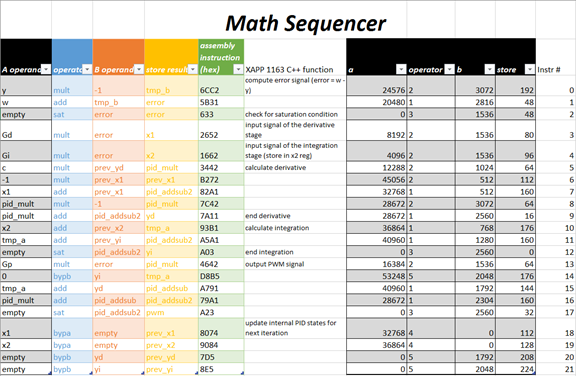Once again, functional simulation indicates there are no functional or dynamic range differences found between the Simulink golden reference model and the Math Sequencer.

Figure 4. Simulink versus Math Sequencer Simulation Results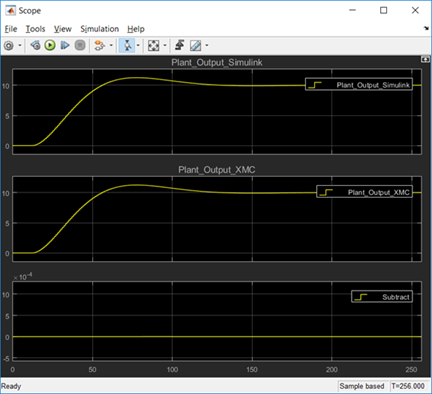As demonstrated in the following figure, by applying two HLS #pragma instructions to the C++ code, the following PL implemented results in the Clock Rate = 1∕1.567e–9 = 638.2 MHz clock; Sample Rate = 1∕(83 × 1.567e–9) = 7.7 MSPS.

Figure 5. PL Implemented Results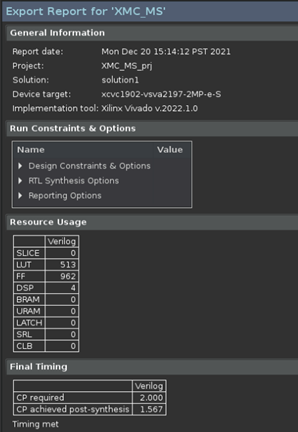Figure 6. Math Sequencer Implementation Results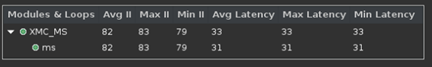The advantage of a Math Sequencer is that it can easily change the operators based on the algorithm requirements. For example, a division operator or a square root function can be added to calculate the magnitude. Also, the Math Sequencer can change the instruction sequence at a later date without changing the hardware implementation. Further, the Math Sequencer can reduce resources at the expense of latency. Direct comparison of the HLS Toolbox and C++ Math Sequencer PID control loop implementations is demonstrated in the following table.

Table 1. Resources, Latency, Clock Frequency, and Sample Rate Comparison
DSP LUTs FFs Block RAM Latency (Clocks) Clock (MHz) Sample Rate (MSPS)
VMC HLS Toolbox 5 565 505 0 69 472 6.8
C++ Math Sequencer 4 513 962 0 83

638.2

7.7